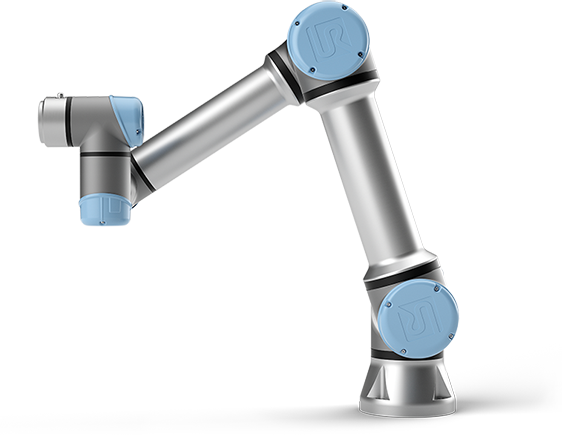Reduce quality of an imported MeshRegion

Importing STL files using Import results in a MeshRegion composed by the triangulation of the original file, and a similar ByteCount as the file in disk.

When trying to render interactive graphics using Manipulate, the time needed to render the visualization of the object is very high, not being able to be done in real time.

Is there a way to reduce the quality/size of the MeshRegion within Mathematica to reduce the size of the object and improve Manipulation speed?

I have tried using DiscretizeRegion and DiscretizeGraphics on the imported object, but it seems it does not work on MeshRegion.

I would like to find a way to do this in Mathematica, without having to rely on a CAD program to import and export the file again with a lower triangulation quality.

EDIT:

Here is an example of an object:• Very good question. However, this is typically a very hard problem. As far as I know, Mathematica does not provide any solution for that. Feb 18 '20 at 19:56
• We can propose an algorithm, but we need an example of mesh. Feb 18 '20 at 21:15
• Edit with an example of the STL file. Feb 18 '20 at 22:08
• @HenrikSchumacher I think this might be possible via sampling like points = RandomPoint[Import["Link.STL"], 1000] and then using your Voronoi code. Feb 20 '20 at 16:38
• @VitaliyKaurov Oh, interesting. I completely forgot about that one. Hmmm... Feb 20 '20 at 17:12

Decimation of mesh is not a trivial task if you want to preserve the visual features of the original mesh. If you want to tap into the efforts of people that have been working on the problem for many years, you could use the freely available MeshLab to perform the decimation with a script driven from Mathematica. Here is one possible workflow (Note: Uncompress to see the underlying MeshLab mlx script that drives the decimation):

SetDirectory[NotebookDirectory[]];
createDeciScript[deci_ : 0.1] :=
StringTemplate[
Uncompress[Import["https://pastebin.com/raw/Fsu2RNLt", "Text"]]][<|
"d" -> deci|>]
fileName = "deci.mlx";
file = OpenWrite[fileName];
WriteString[file, createDeciScript[0.1]];
Close[file];
cmd = "\"C:\\Program Files\\VCG\\MeshLab\\meshlabserver.exe\" -i \
Run[cmd];The image on the right has 10x fewer triangles than the image on the left.• @LCarvalho Interesting. That definitely appears to be the logo. I was just treating the source material as a Bag-Of-Triangles to be decimated. Oct 14 '20 at 20:29
• meshlabserver.exe was discontinued in favor of a python interface, see here: stackoverflow.com/questions/65825861/… Aug 15 '21 at 8:38...if nothing else a very poor man's solution for simple visual in an interactive interface is just random sampling of points (i know it is far from spectacular :-) but is very light):

pts=RandomPoint[obj,1000];
obj//ByteCount
Graphics3D[Point[pts]]//ByteCount

1808792

24792

Also I just would like to outline a strategy for this, and explain why it might be a good approach. I cannot run complete wonderful code by @HenrikSchumacher (tech difficulties), but people here I bet can figure it out. So now if we think of somehow roughening polygons to lower resolution we might run into a problem, depending on a case, and this case is a good example. Let's see how points are distributed in the original .STL object:

Graphics3D[MeshPrimitives[obj, 0]]Do you see how many are dense in one region and sparse in another? So some blunt approach of locally down-sampling polygons might require a very complicated algorithm, but still be inefficient somehow due to highly non-uniform down-sampling. Now we can think of another approach. We have ability to randomly point-sample any high-res region:

pts=RandomPoint[obj,200];

and you can see how points now appear in the regions with low density of points in the original mesh:

Show[
HighlightMesh[RegionBoundary[obj],{Style[2,Opacity[0.2]],Style[1,Opacity[0.4]]}],
Graphics3D[{Red,Sphere[pts,1]}],
Boxed->True,Axes->True]Now you can run wonderful code by @HenrikSchumacher and others seen in posts about VORONOI and GEODESICS to be ready to go, or maybe you can also think of applying a spline approach as an interesting alternative discussed HERE - but this might need more jumping through the hoops in terms of proper data for BSplineFunction.

• It wasn't the preferred solution for the original question, but I have come back to find this useful in a different case. Thank you! Jun 18 '20 at 10:19
• I have been struggling to apply those codes to this example. How should I input this pts to the Voronoi and Geodesics code? Could you show how the last part is performed on this example? Jun 18 '20 at 11:00
• Could this perform better than computing a Concave Hull of the randomly selected points? Jun 23 '20 at 14:15

I have been trying to do this calculating a Concave Hull using this approach:

pts = RandomPoint[obj, 10000];
tetrahedra = Level[MeshPrimitives[DelaunayMesh[pts], 3], {-3}];
alphashape[rmax_] := Pick[tetrahedra, radii, r_ /; r < rmax]
faces[tetras_] := Flatten[
tetras /. {a_, b_, c_, d_} :> {{a, b, c}, {a, b, d}, {a, c, d}, {b, c, d}}, 1];
externalfaces[faces_] := Cases[Tally[Sort /@ faces], {face_, 1} :> face];